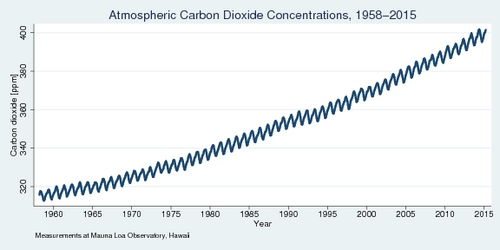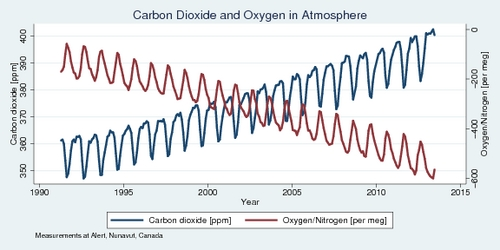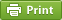Werner's Blog — Opinion, Analysis, Commentary
Fossil fuels and declining oxygen in our atmosphere

If there was a smoking gun to prove the anthropogenic forcing of climate change, what would it be? We have witnessed a large increase in concentrations of carbon dioxide in our atmosphere since the days of industrialization. Is that enough to prove that it is caused by burning of fossil fuel? It turns out that a little bit of chemistry makes the case rather convincingly, after all.

Earth's atmosphere contains 78.1% nitrogen and 20.9% oxygen, as well as traces of other gases including carbon dioxide. The increase in carbon dioxide concentrations since the beginning of industrialization has been identified as the key culprit in climate change. The continuous monitoring of concentrations at the Mauna Loa Observatory in Hawaii, shown in the diagram below, shows the rapid increase over nearly sixty years. In 2014, carbon dioxide concentrations topped 400 parts per million (ppm) for the first time.Burning fossil fuels (carbon) requires oxygen: $$\mathrm{C}+\mathrm{O}_2 \rightarrow\mathrm{CO}_2$$. By molecular weights, 1 gram of carbon combines with 2.67 grams of oxygen to yield 3.67 grams of carbon dioxide. (The molecular weights of carbon, oxygen, and carbon dioxide are 12, 16, and 44, respectively.) Total carbon dioxide emissions in 2014 amounted to 32 gigatonnes. Therefore, 72.7% of this mass, or 23.3 gigatonnes, is oxygen. The total weight of oxygen in our atmosphere is estimated at 1,080,000 gigatonnes. Dividing the two numbers and multiplying by one million, the reduction in our atmosphere's oxygen concentration due to carbon dioxide generation comes to 22 "per meg". As it turns out, that is just about what new scientific methods have found as the annual decrease in oxygen levels.

The Scripps O2 Program at the University of California at San Diego has documented a decrease in our atmosphere's concentration of oxygen since the late 1980s. The method used by the Scripps Institution of Oceanography is interferometry, which exploits the refractive properties of different wavelengths to measure the oxygen/nitrogen ratio in the atmospehere. Specifically, changes in oxygen concentrations are obtained by comparing the $$\mathrm{O}_2/\mathrm{N}_2$$ mole ratio of an air sample with that of a reference sample captured in the mid-1980s, which is stored in a laboratory at Scripps. The ratio that is measured is defined as $\delta\left(\frac{\mathrm{O}_2}{\mathrm{N}_2}\right)=10^6\cdot\left[ \frac{(\mathrm{O}_2/\mathrm{N}_2)_{\mathrm{sample}}}{(\mathrm{O}_2/\mathrm{N}_2)_{\mathrm{reference}}}-1\right]$ The measure $$\delta$$ is expressed in "per meg", where one "per meg" is one molecule of oxygen in a million. Using the "per meg" measure for oxygen is preferred over the ppm measure, but they are roughly equivalent at 4.77 meg to 1 ppm. The research, under the direction of Prof. Ralph Keeling, shows that oxygen concentrations are currently declining at roughly 19–20 per meg per year, or about 4 ppm per year.

The diagram below shows oxygen and carbon dioxide measurements from the observation station in Alert, Nunavut, at 82°N latitude. The data series only starts in 1991, but the two trends are nearly perfectly negatively correlated. A 1 ppm increase in the atmosphere's carbon dioxide concentrations results in a 10 per meg decrease in the atmosphere's oxygen level.The data shows that the oxygen level is decreasing at a rate of about 20 per meg per year over the last quarter century. Calculations above had suggested that 32 gigatonnes of carbon dioxide were added to the atmosphere in total in 2014, which amounts to about 6 ppm. However, measurements went up only by about 2 per year. Where did all the extra carbon dioxide go? At least half, or perhaps two-thirds, of carbon dioxide emissions is absorbed by mother nature, mostly the oceans. Without that help, climate change would be even more dramatic. The oceans pay a price for all that extra carbon dioxide, which forms carbonic acid with water and makes the ocean more acidic, which in turn damages coral reefs and marine life.

The decrease in our atmosphere's oxygen is proof that the increase in carbon dioxide in our atmosphere is anthropogenic. It is the smoking gun in the debate about the origin of climate change.

References:

Posted on Monday, June 1, 2015 at 21:15 — #EnvironmentRecent Blog Entries

Topics

Months

Subscribe to RSS feed© 2023  Prof. Werner Antweiler, University of British Columbia.